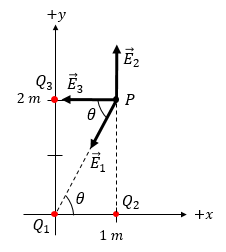# Point charge Q_1= -1.0 nC is at the origin and point charge Q_2= +1 nC is on the x-axis at...

## Question:

Point charge {eq}Q_1= -1.0{/eq} nC is at the origin and point charge {eq}Q_2= +1{/eq} nC is on the x-axis at {eq}(1\text{m},0,0){/eq}. A third charge {eq}Q_3= -2{/eq} nC is on the y axis at {eq}(0,2\text{m},0){/eq}. Observation point P is at {eq}(1\text{m},2\text{m},0){/eq}.

a) Find the electric field {eq}E_1{/eq} due to {eq}Q_1{/eq} at P.

b) Find the electric field {eq}E_2{/eq} due to {eq}Q_2{/eq} at P.

c) Find the electric field {eq}E_3{/eq} due to {eq}Q_3{/eq} at P.

d) Find the total electric field due to all the charges at point P. Also find its magnitude and direction.

## Electric Field Due to Multiple Charges:

In a system consisting of multiple point charges, the total (net) electric field at a point due to these charges is the vector sum of the individual electric field contributions. Electric field ({eq}\vec{E}{/eq}) is a vector quantity, i.e., it has a magnitude and direction. Therefore, in solving for the electric field due to a point charge, it is necessary to express the electric field vector in terms of its vector components. In a 2-dimensional Cartesian coordinate system, we can express {eq}\vec{E}{/eq} as {eq}\vec{E} = E_x \ \hat{i} + E_y \ \hat{j} = E \ cos \ \theta \ \hat{i} + E \ sin \ \theta \ \hat{j}{/eq}.#### Part a:

The reference angle is {eq}\theta = tan^{-1} \ (2 \ m/1 \ m) = 63.4^{\circ}{/eq}. As a convention, we measure...

Become a Study.com member to unlock this answer! Create your account